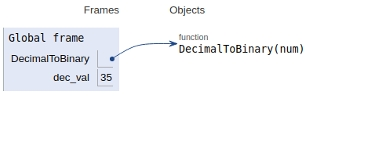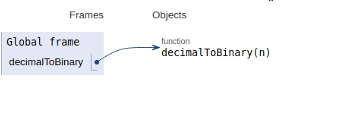# Python program to convert decimal to binary number

In this article, we will learn about the solution and approach to solve the given problem statement.

## Problem statement

Given a number we need to convert into a binary number.

## Approach 1 − Recursive Solution

DecToBin(num):
if num > 1:
DecToBin(num // 2)
print num % 2

### Example

def DecimalToBinary(num):
if num > 1:
DecimalToBinary(num // 2)
print(num % 2, end = '')
# main
if __name__ == '__main__':
dec_val = 35
DecimalToBinary(dec_val)

### Output

100011

All the variables and functions are declared in the global scope as shown below −## Approach 2 − Built-in Solution

### Example

Live Demo

def decimalToBinary(n):
return bin(n).replace("0b", "")
# Driver code
if __name__ == '__main__':
print(decimalToBinary(35))

### Output

100011

All the variables and functions are declared in the global scope as shown below −## Conclusion

In this article, we learnt about the approach to convert a decimal number to a binary number.